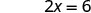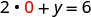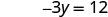# 4.3: Graph with Intercepts

•• OpenStax
• OpenStax
$$\newcommand{\vecs}{\overset { \rightharpoonup} {\mathbf{#1}} }$$ $$\newcommand{\vecd}{\overset{-\!-\!\rightharpoonup}{\vphantom{a}\smash {#1}}}$$$$\newcommand{\id}{\mathrm{id}}$$ $$\newcommand{\Span}{\mathrm{span}}$$ $$\newcommand{\kernel}{\mathrm{null}\,}$$ $$\newcommand{\range}{\mathrm{range}\,}$$ $$\newcommand{\RealPart}{\mathrm{Re}}$$ $$\newcommand{\ImaginaryPart}{\mathrm{Im}}$$ $$\newcommand{\Argument}{\mathrm{Arg}}$$ $$\newcommand{\norm}{\| #1 \|}$$ $$\newcommand{\inner}{\langle #1, #2 \rangle}$$ $$\newcommand{\Span}{\mathrm{span}}$$ $$\newcommand{\id}{\mathrm{id}}$$ $$\newcommand{\Span}{\mathrm{span}}$$ $$\newcommand{\kernel}{\mathrm{null}\,}$$ $$\newcommand{\range}{\mathrm{range}\,}$$ $$\newcommand{\RealPart}{\mathrm{Re}}$$ $$\newcommand{\ImaginaryPart}{\mathrm{Im}}$$ $$\newcommand{\Argument}{\mathrm{Arg}}$$ $$\newcommand{\norm}{\| #1 \|}$$ $$\newcommand{\inner}{\langle #1, #2 \rangle}$$ $$\newcommand{\Span}{\mathrm{span}}$$$$\newcommand{\AA}{\unicode[.8,0]{x212B}}$$

##### Learning Objectives

By the end of this section, you will be able to:

• Identify the x- and y- intercepts on a graph
• Find the x- and y- intercepts from an equation of a line
• Graph a line using the intercepts
##### Note

Before you get started, take this readiness quiz.

1. Solve: $$3\cdot 0+4y=−2$$.
If you missed this problem, review Exercise 2.2.13.

## Identify the x- and y- Intercepts on a Graph

Every linear equation can be represented by a unique line that shows all the solutions of the equation. We have seen that when graphing a line by plotting points, you can use any three solutions to graph. This means that two people graphing the line might use different sets of three points.

At first glance, their two lines might not appear to be the same, since they would have different points labeled. But if all the work was done correctly, the lines should be exactly the same. One way to recognize that they are indeed the same line is to look at where the line crosses the x- axis and the y- axis. These points are called the intercepts of the line.

##### INTERCEPTS OF A LINE

The points where a line crosses the x- axis and the y- axis are called the intercepts of a line.

Let’s look at the graphs of the lines in Figure $$\PageIndex{1}$$.Figure $$\PageIndex{1}$$: Examples of graphs crossing the x-negative axis.

First, notice where each of these lines crosses the x negative axis. See Figure $$\PageIndex{1}$$.

Table $$\PageIndex{1}$$
Figure The line crosses the x-axis at: Ordered pair of this point
Figure (a) 3 (3,0)
Figure (b) 4 (4,0)
Figure (c) 5 (5,0)
Figure (d) 0 (0,0)

Do you see a pattern?

For each row, the y- coordinate of the point where the line crosses the x- axis is zero. The point where the line crosses the x- axis has the form (a,0) and is called the x- intercept of a line. The x- intercept occurs when y is zero. Now, let’s look at the points where these lines cross the y- axis. See Table $$\PageIndex{2}$$.

Table $$\PageIndex{2}$$
Figure The line crosses the x-axis at: Ordered pair of this point
Figure (a) 6 (0,6)
Figure (b) −3 (0,−3)
Figure (c) −5 (0,5)
Figure (d) 0 (0,0)

What is the pattern here?

In each row, the x- coordinate of the point where the line crosses the y- axis is zero. The point where the line crosses the y- axis has the form (0,b) and is called the y- intercept of the line. The y- intercept occurs when x is zero.

##### X- INTERCEPT AND Y- INTERCEPT OF A LINE

The x- intercept is the point (a,0) where the line crosses the x- axis.

The y- intercept is the point (0,b) where the line crosses the y- axis.Figure $$\PageIndex{2}$$
##### Exercise $$\PageIndex{1}$$

Find the x- and y- intercepts on each graph.Figure $$\PageIndex{3}$$

(a) The graph crosses the x- axis at the point (4,0). The x- intercept is (4,0).
The graph crosses the y- axis at the point (0,2). The y- intercept is (0,2).

(b) The graph crosses the x- axis at the point (2,0). The x- intercept is (2,0)
The graph crosses the y- axis at the point (0,−6). The y- intercept is (0,−6).

(c) The graph crosses the x- axis at the point (−5,0). The x- intercept is (−5,0).
The graph crosses the y- axis at the point (0,−5). The y- intercept is (0,−5).

##### Exercise $$\PageIndex{2}$$

Find the x- and y- intercepts on the graph.x- intercept: (2,0); y- intercept: (0,−2)

##### Exercise $$\PageIndex{3}$$

Find the x- and y- intercepts on the graph.x- intercept: (3,0), y- intercept: (0,2)

## Find the x- and y- Intercepts from an Equation of a Line

Recognizing that the x- intercept occurs when y is zero and that the y- intercept occurs when x is zero, gives us a method to find the intercepts of a line from its equation. To find the x- intercept, let y=0 and solve for x. To find the y- intercept, let x=0 and solve for y.

##### X- AND Y- INTERCEPTS FROM THE EQUATION OF A LINE

Use the equation of the line. To find:

• the x- intercept of the line, let y=0 and solve for x.
• the y- intercept of the line, let x=0 and solve for y.
##### Exercise $$\PageIndex{4}$$

Find the intercepts of 2x+y=6.

We will let y=0 to find the x- intercept, and let x=0 to find the y- intercept. We will fill in the table, which reminds us of what we need to find.To find the x- intercept, let y=0.Let y = 0.Simplify.The x-intercept is (3, 0) To find the y-intercept, let x = 0.Let x = 0.Simplify.The y-intercept is (0, 6)
The intercepts are the points (3,0) and (0,6) as shown in Table $$\PageIndex{4}$$.
Table $$\PageIndex{4}$$
2x+y=6
x y
3 0
0 6
##### Exercise $$\PageIndex{5}$$

Find the intercepts of 3x+y=12.

x- intercept: (4,0), y- intercept: (0,12)

##### Exercise $$\PageIndex{6}$$

Find the intercepts of x+4y=8.

x- intercept: (8,0), y- intercept: (0,2)

##### Exercise $$\PageIndex{7}$$

Find the intercepts of 4x–3y=12.

 To find the x-intercept, let y = 0.Let y = 0.Simplify.The x-intercept is (3, 0) To find the y-intercept, let x = 0.Let x = 0.Simplify.The y-intercept is (0, −4)
Table $$\PageIndex{5}$$

The intercepts are the points (3, 0) and (0, −4) as shown in the following table.

 4x−3y=12 x y 3 0 0 −4
##### Exercise $$\PageIndex{8}$$

Find the intercepts of 3x–4y=12.

x- intercept: (4,0), y- intercept: (0,−3)

##### Exercise $$\PageIndex{9}$$

Find the intercepts of 2x–4y=8.

x- intercept: (4,0), y- intercept: (0,−2)

## Graph a Line Using the Intercepts

To graph a linear equation by plotting points, you need to find three points whose coordinates are solutions to the equation. You can use the x- and y- intercepts as two of your three points. Find the intercepts, and then find a third point to ensure accuracy. Make sure the points line up—then draw the line. This method is often the quickest way to graph a line.

##### Exercise $$\PageIndex{10}$$: How to Graph a Line Using Intercepts

Graph –x+2y=6 using the intercepts.##### Exercise $$\PageIndex{11}$$

Graph x–2y=4 using the intercepts.##### Exercise $$\PageIndex{12}$$

Graph –x+3y=6 using the intercepts.The steps to graph a linear equation using the intercepts are summarized below.

##### GRAPH A LINEAR EQUATION USING THE INTERCEPTS.
1. Find the x- and y- intercepts of the line.
• Let y=0 and solve for x
• Let x=0 and solve for y.
2. Find a third solution to the equation.
3. Plot the three points and check that they line up.
4. Draw the line.
##### Exercise $$\PageIndex{13}$$

Graph 4x–3y=12 using the intercepts.

Find the intercepts and a third point.We list the points in Table $$\PageIndex{7}$$ and show the graph below.

 4x−3y=12 x y (x,y) 3 0 (3,0) 0 −4 (0,−4) 6 4 (6,4)
Table $$\PageIndex{7}$$##### Exercise $$\PageIndex{14}$$

Graph 5x–2y=10 using the intercepts.##### Exercise $$\PageIndex{15}$$

Graph 3x–4y=12 using the intercepts.##### Exercise $$\PageIndex{16}$$

Graph y=5x using the intercepts.This line has only one intercept. It is the point (0,0).

To ensure accuracy we need to plot three points. Since the x- and y- intercepts are the same point, we need two more points to graph the line.See Table $$\PageIndex{8}$$.

 y=5x x y (x,y) (0,0) (1,5) −1 −5 (−1,−5)
Table $$\PageIndex{8}$$

Plot the three points, check that they line up, and draw the line.##### Exercise $$\PageIndex{17}$$

Graph y=4x using the intercepts.##### Exercise $$\PageIndex{18}$$

Graph y=−x the intercepts.## Key Concepts

• Find the x- and y- Intercepts from the Equation of a Line
• Use the equation of the line to find the x- intercept of the line, let y=0 and solve for x.
• Use the equation of the line to find the y- intercept of the line, let x=0 and solve for y.
• Graph a Linear Equation using the Intercepts
1. Find the x- and y- intercepts of the line.
Let y=0 and solve for x.
Let x=0 and solve for y.
2. Find a third solution to the equation.
3. Plot the three points and then check that they line up.
4. Draw the line.
• Strategy for Choosing the Most Convenient Method to Graph a Line:
• Consider the form of the equation.
• If it only has one variable, it is a vertical or horizontal line.
x=a is a vertical line passing through the x- axis at a
y=b is a horizontal line passing through the y- axis at b.
• If y is isolated on one side of the equation, graph by plotting points.
• Choose any three values for x and then solve for the corresponding y- values.
• If the equation is of the form ax+by=c, find the intercepts. Find the x- and y- intercepts and then a third point.

## Glossary

intercepts of a line
The points where a line crosses the x- axis and the y- axis are called the intercepts of the line.
x- intercept
The point (a,0) where the line crosses the x- axis; the x- intercept occurs when y is zero.
y-intercept
The point (0,b) where the line crosses the y- axis; the y- intercept occurs when x is zero.

This page titled 4.3: Graph with Intercepts is shared under a CC BY license and was authored, remixed, and/or curated by OpenStax.